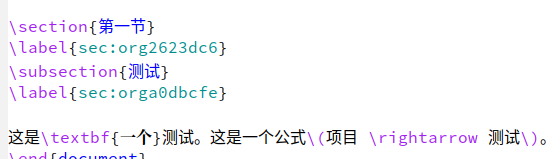# 不用零宽空格在 org-mode 中标记中文的办法

(defun eli-strip-ws-maybe (text _backend _info)
(let* ((text (replace-regexp-in-string
"\$$\\cc\$$ *\n *\$$\\cc\$$"
"\\1\\2" text));; remove whitespace from line break
;; remove whitespace from org-emphasis-alist'
(text (replace-regexp-in-string "\$$\\cc\$$ \$$.*?\$$ \$$\\cc\$$"
"\\1\\2\\3" text))
;; restore whitespace between English words and Chinese words
(text (replace-regexp-in-string "\$$\\cc\$$\$$\\(?:<[^>]+>\$$?[a-z0-9A-Z-]+\$$?:<[^>]+>\$$?\\)\$$\\cc\$$"
"\\1 \\2 \\3" text))
(text (replace-regexp-in-string "\$$\\cc\$$ ?\$$\\\\[^{}()]*?\$$\$$\\cc\$$"
"\\1 \\2 \\3" text)))
text))


#+title: Test

#+LATEX_CLASS: beamer
#+OPTIONS: H:2 toc:t num:t
#+startup: beamer

* 第一节
** 测试\begin{document}

\maketitle
\begin{frame}{Outline}
\tableofcontents
\end{frame}

\section{第一节}
\label{sec:orgb20d632}
\begin{frame}[label={sec:orga84fe28}]{测试}

\end{frame}
\end{document}


#+title: Test

#+LATEX_CLASS: beamer
#+OPTIONS: H:2 toc:t num:t
#+startup: beamer

* 第一节
** 测试



\begin{document}

\maketitle
\begin{frame}{Outline}
\tableofcontents
\end{frame}

\section{第一节}
\label{sec:org4df4d97}
\begin{frame}[label={sec:orgff7d1eb}]{测试}

\end{frame}
\end{document}


(defun eli-strip-ws-maybe (text _backend _info)
(let* ((text (replace-regexp-in-string
"\$$\\cc\$$ *\n *\$$\\cc\$$"
"\\1\\2" text));; remove whitespace from line break
;; remove whitespace from org-emphasis-alist'
(text (replace-regexp-in-string "\$$\\cc?\$$ \$$.*?\$$ \$$\\cc\$$"
"\\1\\2\\3" text))
;; restore whitespace between English words and Chinese words
(text (replace-regexp-in-string "\$$\\cc\$$\$$\\(?:<[^>]+>\$$?[a-z0-9A-Z-]+\$$?:<[^>]+>\$$?\\)\$$\\cc\$$"
"\\1 \\2 \\3" text))
(text (replace-regexp-in-string "\$$\\cc\$$ ?\$$\\\\[^{}()]*?\$$\$$\\cc\$$"
"\\1 \\2 \\3" text)))
text))


edit: 再次更新

1 个赞